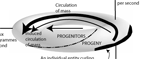﻿ Synopsis | Engenetics: The science of species
• THE SYNOPSIS (go here for the full exposition of the theory)

• Begin by offering definitions for biology and ecology. State and outline a rigorous biological-ecological model. Argue that basic terms are in dispute, and that there is a lack of agreement on core variables. Describe the Brassica rapa experiment. Discuss the origins and consequences of the split between the biological and the physical sciences. Darwin lays the foundations. Maxwell lays more foundations. Boyle, Mayer and the energy doctrine. The significance for biology of Green’s theorem and the planimeter. State the constraints upon biological populations. Note similarities between Mayer, Joule, Maxwell and Darwin. Energy, metabolism, genes and the equilibrium distribution. That biological populations induce biological behaviour and natural selection. Applying the vector calculus—gradient theorem of line integrals, Green’s theorem, Stokes’ theorem and Gauss’ theorem—to natural selection. Mass, growth, entropy and natural selection. Presenting the Euler and Gibbs-Duhem population equations. Deriving the four ‘laws’ of biology. Deriving the four ‘maxims’ of ecology. Using polar coordinates to define generation length. The cycle of the generations and the Liouville and Helmholtz theorems. The uniqueness of populations and species. Demonstrating the Lagrange multiplier for biology. Applying the model to Brassica rapa. Confirming the original definitions. Confirming the vector basis for natural selection, competition and evolution. Final proof that a population free from Darwinian competition and evolution is impossible. Examining the mass-energy a biological population uses. Using the Einstein theory of relativity to create both (a) a general theory for biology; and (b) a quantum biology.

G  O    T  O    R  E  L  A  T  I  V  I  T  YLAW 1: The law of existence
n ≥ 1; δW = (δQ - dU) > 0; m → ∞; > 0

LAW 2: The law of equivalence
[(δW1 = δW2) ∧ (δW2 = δW3)] ⇒ (δW1 = δW3)

LAW 3: The law of diversity
A → 0; FM

LAW 4: The law of reproduction
[(dm̅/dt ≤ 0) ∧ ( > 0)] ⇒ [(dn/dt ≥ 0) ∧ (dA/dt > 0)]MAXIM 1: The maxim of dissipation
[Darwin's theory of competition]
M = nm̅;   ∫dm < 0;   ∇ • M → 0

MAXIM 2: The maxim of number
∇ • H = H/n =

MAXIM 3: The maxim of succession
[Darwin's theory of evolution]
∇ x M = ∂/∂t - ∂n/∂t

MAXIM 4: The maxim of apportionment
∇ x H = ∂/∂t - ∂n/∂t - ∂V/∂t